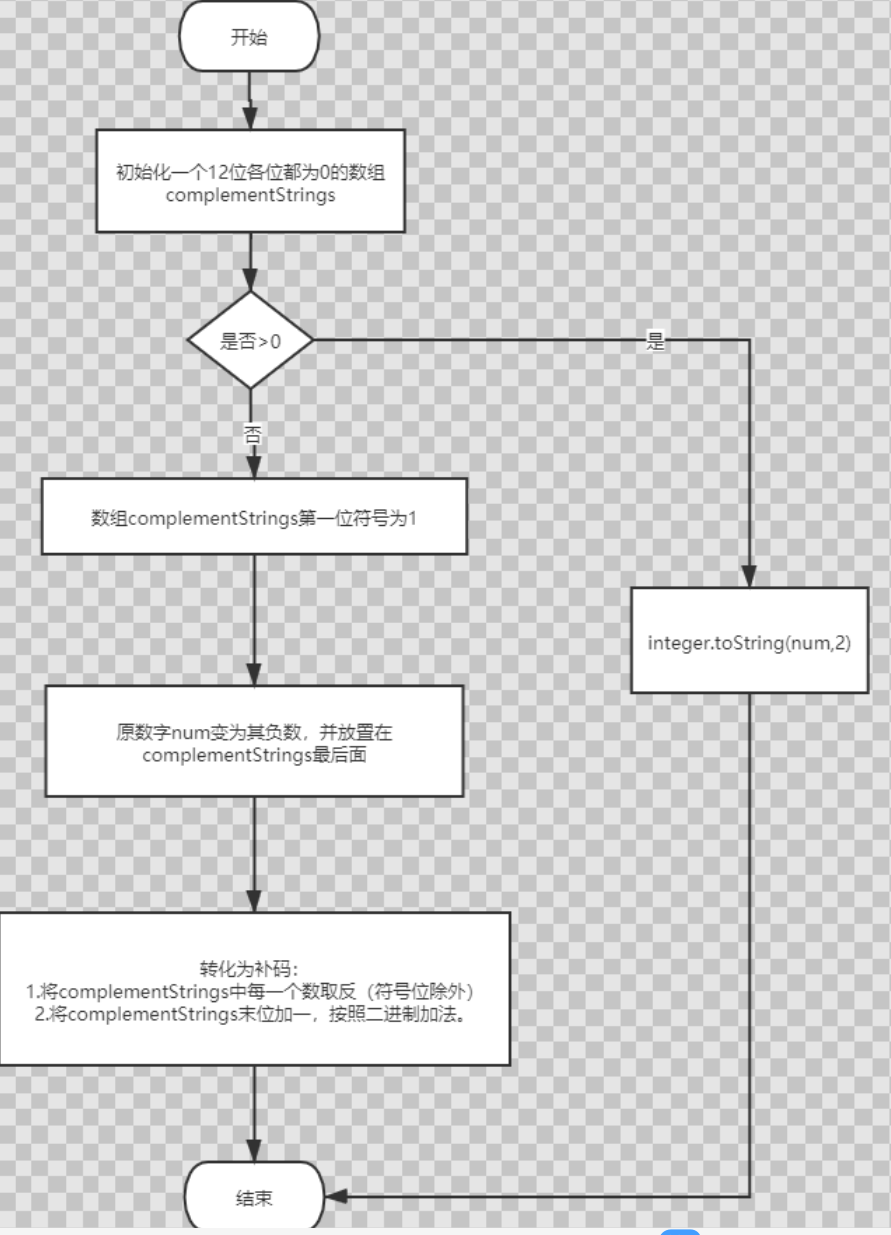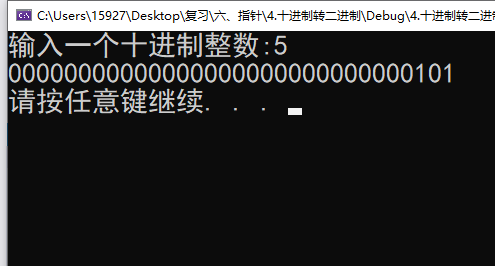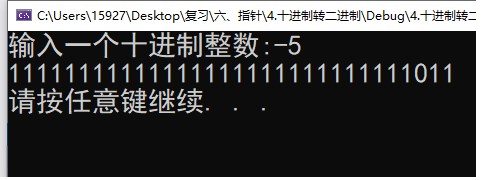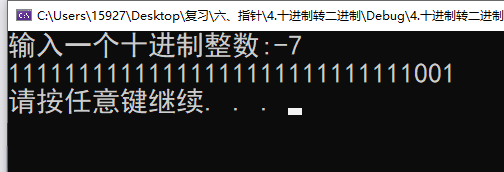• 二进制补码转换十进制整数
千次阅读
2021-04-18 05:07:24

转载：http://blog.xdnice.com/blog40543i59178.html

matlab提供了一个系统函数dec2bin,可以用来进行十进制数的二进制转换,不过功能有限!

在matlab中键入 help dec2bin,如下:

DEC2BIN Convert decimal integer to a binary string.

DEC2BIN(D) returns the binary representation of D as a string.

D must be a non-negative integer smaller than 2^52.

DEC2BIN(D,N) produces a binary representation with at least

N bits.

Example

dec2bin(23) returns '10111'

可见,这个函数只能转换整数,对非整数就无能为力了.因此,自己编写了matlab小程序,顺便也贴出来,希望对需要的人能有所帮助!^_^

1.小数转换为二进制数

输入参数有2个,一个是输入的小数(小于1),一个是指定转换后的二进制位数!程序源码如下:

y=dectobin(innum,N)

%十进制小数转换为二进制数

%输入参数为innum和N

%innum为输入的十进制小数

%N为指定转换后二进制的位数

if (innum>1)|(N == 0)%判断输入的有效性

disp('error!');

return;

end

count=0;

tempnum=innum;

record=zeros(1,N);

while(N)

count=count+1;%长度小于N

if(count>N)

N=0;

%         return;

end

tempnum=tempnum*2;%小数转换为二进制,乘2取整

if tempnum>1

record(count)=1;

tempnum=tempnum-1;

elseif(tempnum==1)

record(count)=1;

N=0;%stop loop

else

record(count)=0;

end

end

y=record;

2.如果要转换整数,则直接调用matlab的dec2bin即可!

3.对于大于1的非整数,可以利用将其分为整数部分和小数部分的方法来处理:

利用matlab的floor函数可以对输入的数(设为innum)向下取整,然后利用innum-floor(innum)就可以得到小数部分,调用上面的函数就可以得到其二进制表达式!

注意,这里有一个小问题,就是dec2bin函数的返回值是一个char array,所以,我们应该先将其转换为double array!可以利用double()进行强制类型转换,得到ASCII码值,减去48就可以得到double array类型的0,1序列!

程序代码如下:

[num,numint,numf]=dectobin1(innum,N)

%十进制数转换为二进制数

%输入为十进制数innum,以及小数部分的位数N

%输出为三个参数num,numint,numf

%num为输出的二进制形式

%numint为整数部分的二进制表达式

%numf为小数部分的二进制表达式

sep=5;%整数和小数部分的分隔符

if(mod(innum,1)==0)%判断输入是否为整数,mod为取余函数

numint=dec2bin(innum);

numint=double(numint)-48;

numf=zeros(1,N);

num=[numint,sep,numf];

return

end;

%输入为非整数的情况

nint=floor(innum);%整数部分

nf=innum-nint;%小数部分

res_nint=dec2bin(nint);

res_nint=double(res_nint)-48;

res_nf=dectobin(nf,N);

numint=res_nint;

numf=res_nf;

num=[numint,sep,numf];

其中的dectobin函数就是最上面的小数转二进制函数!

4.似乎已经大功告成了,是吗?NO,还有一个问题,那就是负数的情况,在这里,我们采用补码的方式,如果输入为正,则二进制表达式不变,如果为负,则按位取反并加1.因此,需要对上面的函数加上一个负数判断以及补码转换的功能.最终的表示结果可以采用1QN的格式.补码转换部分程序如下:

[numo,numinto,numfo]=conv(numint,numf,flag)

%二进制数的补码表示

%输入参数为numint整数部分的二进制表达式,numf小数部分二进制表达式,flag负数标志

%输出参数为numo输入的补码,numinto整数部分的补码,numfo小数部分的补码

if (flag==0)

numo=[0,numint,5,numf];%正数

numinto=numint;

numfo=numf;

else%负数,整数和小数部分均进行按位取反并加一

l1=length(numint);

l2=length(numf);

num=[numint,numf];

l=l1+l2;

for i=1:l

if num(i)==1%按位取反

num(i)=0;

else

num(i)=1;

end

end

%取反后加一

temp_l=l;

while(temp_l~=0)

if num(temp_l)==0%最低位为0

num(temp_l)=1;

temp_l=0;%结束循环

else

num(temp_l)=0;

temp_l=temp_l-1;

end

end

%    l1=length(numint);

%    for i=1:l1

%

%        if numint(i)==1%按位取反

%            numint(i)=0;

%        else

%            numint(i)=1;

%        end

%

%    end

%

%    l2=length(numf);

%    for i=1:l2

%

%        if numf(i)==1%按位取反

%            numf(i)=0;

%        else

%            numf(i)=1;

%        end

%

%    end

%

% %取反后加一

%   temp_l1=l1;

%   while(temp_l1~=0)

%     if numint(temp_l1)==0%最低位为0

%         numint(temp_l1)=1;

%         temp_l1=0;%结束循环

%     else

%         numint(temp_l1)=0;

%         temp_l1=temp_l1-1;

%     end

%   end

%

%   %取反后加一

%   temp_l2=l2;

%   while(temp_l2~=0)

%     if numf(temp_l2)==0%最低位为0

%         numf(temp_l2)=1;

%         temp_l2=0;%结束循环

%     else

%         numf(temp_l2)=0;

%         temp_l2=temp_l2-1;

%     end

numinto=num(1:l1);

numfo=num(l1+1:l);

%   numinto=numint;

%   numfo=numf;

numo=[1,numinto,5,numfo];

end

5.最后,用一个main函数连接以上的子函数

[numo,numinto,numfo]=decimal2bin(innum,N)

[num1,numint1,numf1,flag1]=dectobin1(innum,N);

[numo,numinto,numfo]=conv(numint1,numf1,flag1)

更多相关内容
• 这些函数像内置的 MATLAB 函数 BIN2DEC 和 DEC2BIN 一样在二进制字符串和十进制数之间进行转换，但可以容纳负整数（通过二进制补码）和分数正负数（通过二进制补码固定点和字符串中的二进制小数点）。 请注意，许多...matlab
• 在 MATLAB 中计算有符号整数二进制补码matlab
• 先考虑如何将二进制补码转换十进制整数: 若符号位是0, 则该数是正数, 原码等同于补码. 可以由原码(也就是补码)得知代表的正整数. 若符号为是1, 则该数一定是负数, 可按照以下方式转换: 方式一: 先把符号位去掉, ...

最近做的一个工程项目用到了基本的数据转换，代码实现了一遍，供大家和自己参考。

先考虑如何将二进制补码转换为十进制整数:

1. 若符号位是0, 则该数是正数, 原码等同于补码. 可以由原码(也就是补码)得知代表的正整数.
2. 若符号为是1, 则该数一定是负数, 可按照以下方式转换: 方式一: 先把符号位去掉, 把剩下的非符号位取反后得到一个无符号位的二进制序列, 将该二进制序列转换为十进制整数(注意:无符号位二进制序列代表的一定是正数),
将该整数加1得到的数便是原补码代表的整数的绝对值. 如: a = 1011(补码), 011取反得到100, 100代表4, 4+1=5,
|a|=5, a=-5. 方式二: 利用公式: (-1)2^(n-1) + 非符号位代表整数 = 值. 如a =
1011(补码), n = 4, 011代表3, a = -1
2^3 + 3 = -5.

功能函数

int u4complement(BYTE *pData) {
int sign = int(pData >> 7);
if(sign == 0)
return  pow(256, 3)*int(pData) + pow(256, 2)*int(pData) + pow(256, 1)*int(pData) + int(pData);
if(sign == 1)
return -1*(pow(256, 3)*int(pData^0xFF) + pow(256, 2)*int(pData^0xFF) + pow(256, 1)*int(pData ^ 0xFF) + int(pData ^ 0xFF)+1);
}


主函数


#include "pch.h"
#include <iostream>
#include "math.h"

using namespace std;
typedef unsigned char BYTE;
int u4complement(BYTE *pData) ;
int main()
{

BYTE *complete = new BYTE;
complete = 0xFF;
complete = 0xFF;
complete = 0xFF;
complete = 0xFA;
cout << u4complement(complete) << endl;
}

展开全文补码
• 今天一场技术笔试一道编程题难住了我，算出一个十进制数的二进制补码和对应的16进制，由于时间紧张，加上紧张，做的极差，因此mark以下

## 十进制转二进制补码

首先要明确的是：十进制直接转成二进制是转化为二进制原码，正数的原码和补码相同，但负数的原码按位取反末位加一才是补码。
因此流程图大概如下：整个代码我贴在最后

## 主要逻辑代码：

public static String convert(int num) {
StringBuilder resultString = new StringBuilder();
// 初始化补码数组，共12位
String[] complementStrings = new String[] { "0", "0", "0", "0", "0", "0", "0", "0", "0", "0", "0", "0" };
boolean ifNegative = false;
if (num < 0) {
complementStrings = "1";
num = -num;
ifNegative = true;
}
// 加入到补码的数组中，开头从12-strings.length开始
String[] strings = Integer.toString(num, 2).split("");
// 对应在strings数组中的下标
int j = 0;
for (int i = 12 - strings.length; i < 12; i++) {
complementStrings[i] = strings[j];
j++;
}
// 如果为负数，先全部取反,否则直接拼接
if (ifNegative) {
for (int i = 1; i < complementStrings.length; i++) {
if (complementStrings[i].equals("1")) {
complementStrings[i] = "0";
} else {
complementStrings[i] = "1";
}
}
// 送进函数加一
resultString.append(binaryPlusOne(complementStrings, 11, 1));
} else {
resultString.append(strings.toString());
}
return resultString.toString();
}



## 重要代码1：末位加一

为递归算法,递归逻辑为每次将一位执行与进位的加法并拼接，走完整个数组，返回。

	/**
*
* @param strings 二进制数数组
* @param temp 对哪一位执行加法
* @param carry 进位值
*/
private static String binaryPlusOne(String[] strings, int temp, int carry) {
if (temp < 0) {
StringBuilder tempBuilder = new StringBuilder();
for (int i = 0; i < strings.length; i++) {
tempBuilder.append(strings[i]);
}
return tempBuilder.toString();
} else {
// 计算加上进位后的值
int tempInt = Integer.parseInt(strings[temp]) + carry;
// 更新对应位置上的值
strings[temp] = String.valueOf(tempInt % 2);
// 更新进位
carry = (tempInt) / 2;
// 更新坐标
temp--;
}
return binaryPlusOne(strings, temp, carry);
}


## 二进制转16进制

同样也是递归的思想，每次将二进制字符串最后四位进行转化，并拼接，知道拼完为止。

## 重要代码

	/**
* 将二进制转为16进制
*
* @param binaryString
* @param length
* @return
*/
private static String binaryTo16(String binaryString, int length) {
if (length < 4) {
return binaryString;
}
return binaryTo16(binaryString.substring(0, length - 4), length - 4)
+ binaryTo16Map.get(binaryString.substring(length - 4, length));
}


## 总体代码，粘了就能用

输入为一个十进制数（其二进制位数在12位以内）
输出为 12位二进制补码和二进制补码对应的16进制码的拼接（以分号隔开）

# 示例：

输入：-7
输出：111111111001;FF9

import java.util.HashMap;

public class BinaryCodeTransfer {
public final static int totalNumber = 12;
public final static HashMap<String, String> binaryTo16Map = new HashMap<String, String>() {
{
put("0000", "0");
put("0001", "1");
put("0010", "2");
put("0011", "3");
put("0100", "4");
put("0101", "5");
put("0110", "6");
put("0111", "7");
put("1000", "8");
put("1001", "9");
put("1010", "A");
put("1011", "B");
put("1100", "C");
put("1101", "D");
put("1110", "E");
put("1111", "F");
}
};

public static void main(String[] args) {
System.out.println(convert(-7));
}

public static String convertToBase72(int num) {

return Integer.toString(num, 2);
}

public static String convert(int num) {
StringBuilder resultString = new StringBuilder();
// 初始化补码数组，共12位
String[] complementStrings = new String[] { "0", "0", "0", "0", "0", "0", "0", "0", "0", "0", "0", "0" };
boolean ifNegative = false;
if (num < 0) {
complementStrings = "1";
num = -num;
ifNegative = true;
}
// 加入到补码的数组中，开头从12-strings.length开始
String[] strings = Integer.toString(num, 2).split("");
// 对应在strings数组中的下标
int j = 0;
for (int i = 12 - strings.length; i < 12; i++) {
complementStrings[i] = strings[j];
j++;
}
// 如果为负数，先全部取反,否则直接拼接
if (ifNegative) {
for (int i = 1; i < complementStrings.length; i++) {
if (complementStrings[i].equals("1")) {
complementStrings[i] = "0";
} else {
complementStrings[i] = "1";
}
}
// 送进函数加一
resultString.append(binaryPlusOne(complementStrings, 11, 1));
} else {
resultString.append(strings.toString());
}

// 如果大于零直接求出十六进制，否则，放入16进制处理函数中
resultString.append(";").append(binaryTo16(resultString.toString(), 12));
return resultString.toString();
}

/**
*
* @param strings
* @param temp
* @param carry
*/
private static String binaryPlusOne(String[] strings, int temp, int carry) {
if (temp < 0) {
StringBuilder tempBuilder = new StringBuilder();
for (int i = 0; i < strings.length; i++) {
tempBuilder.append(strings[i]);
}
return tempBuilder.toString();
} else {
// 计算加上进位后的值
int tempInt = Integer.parseInt(strings[temp]) + carry;
// 更新对应位置上的值
strings[temp] = String.valueOf(tempInt % 2);
// 更新进位
carry = (tempInt) / 2;
// 更新坐标
temp--;
}
return binaryPlusOne(strings, temp, carry);
}

/**
* 将二进制转为16进制
*
* @param binaryString
* @param length
* @return
*/
private static String binaryTo16(String binaryString, int length) {
if (length < 4) {
return binaryString;
}
return binaryTo16(binaryString.substring(0, length - 4), length - 4)
+ binaryTo16Map.get(binaryString.substring(length - 4, length));
}
}


展开全文补码 java
• 救急孩子，计算机导论作业。程序要求输入一个服127到127之间的十进制整数，输出一个八位的进制整数python
• 十进制整数转换为二进制整数采用”除2取余，逆序排列”法。具体做法是：用2去除十进制整数，可以得到一个商和余数；再用2去除商，又会得到一个商和余数，如此进行，直到商为零时为止，然后把先得到的余数作为二进制...
• 将一个十进制正(负)整数转换为对应的二进制补码(用指针完成 十进制转二进制:1.先判断该整数是正数还是负数 如果是正数则二进制补码首位为1 , 且对应的二进制补码就是原. 如果是 负数 则二进制补码为原码基础上取反且...

# 代码:

#include"stdio.h"
#include"Stdio.h"
int main()
{
int *p;
int x,i;

printf("输入一个十进制整数:");
scanf_s("%d", &x);

p = (int *)malloc(sizeof(int) * 32);//为二进制分配内存空间

if (x < 0)//负数转正  如果为负数 二进制第一位为1 正数则为0
{
*p = 1;
x = -x;
}
else if (x > 0)    *p = 0;
//判断正负    改首位
//-----------------------------------------------------------------------
for(i=31;i>=1;i--)//原码
{
*(p+i) = x % 2;
x = x / 2;
}
//-----------------------------------------------------------------------

//二进制取补码规则;若x为负数则 源码换反码 且反码末位加1
if (*p == 1)//换反码
{
for (i = 1; i <=31; i++)
{
if (*(p+i) == 1)       *(p + i) = 0;
else				   *(p + i) = 1;
}
}

if (*p == 1)//负数
{
if (*(p+31) == 0)	*(p + 31) = 1;//最后一位为0 则直接加1
else if (*(p + 31) == 1)      //最后一位为1   进位
{
for (i = 1; i <= 31; i++)
{
if (*(p + 31) + 1 == 2 )//逢2进1
{
*(p + 31) = 0;
*(p +30) += 1;
}
else if (i > 0 && *(p + i) == 2)
{
*(p + i) = 0;
*(p + i+1) += 1;
}
}
}
}

//-----------------------------------------------------------------------
for (i = 0; i <= 31; i++)//输出
{
printf("%d", *(p + i));
}
printf("\n");

system("pause");
return 0;
}


# 运行测试:

#### 测试: 5 结果:000000000000000000000000000101#### 测试: -5 结果:11111111111111111111111111111011#### 测试: -7 结果:11111111111111111111111111111011初学阶段,算法可能有问题,不妥的多提意见…

参考资料:

展开全文补码
• 二进制整数转换十进制整数 【问题描述】 编写程序，输入字符串形式的进制整数（长度为16位，采用补码表示），把它转换为十进制整数，输出该十进制整数补码首位表示符号，0代表正数，1代表负数。 需要注意的是...负数的补码代码实现
• 二进制补码转为十进制整数 package kevin.demo; import java.util.Arrays;import java.util.Scanner;public class Hello { public static void main(String[] args) { Scanner in = new Scanner(System.in); String ...
• 前置知识：二进制与十进制相互转换、原码反码补码移码十进制整数二进制补码用基本的方法：通过十进制转化为二进制，二进制转为原码，再转反码，再转补码。。。。 详见：...
• ## 二进制补码详解

千次阅读 2022-03-27 10:29:49
微处理器用二进制补码系统表示有符号整数，因为它可以将减法运算转换为对减数的补数的加法运算，详解如下。系统安全
• C++学习记录：将十进制转换二进制数（补码形式） 0、以下操作前提：不动符号位 基本运算： 1、正数的补码 等于 原码； 2、负数的补码 等于 原码取反，末位再加一； 推论： 3、补码补码 等于 原码； 4、反码的...
• 二进制补码进制补码及其内的运算——关于补码的一点学习
• 定义函数to_complement(n, bits)，对于给定的一个整数n和一个正整数bits，该函数输出整数 n 的bits位二进制补码表示。 例如： to_complement(5,8)输出00000101 to_complement(-5,8)输出11111011...python
• 使用python进行十进制二进制相互转换 python中进制转换有专门的函数，如hex,int,oct,bin.见下图. 不过如果我将一个负数使用bin转换为2进制，得到的并不是补码形式。如： >>> bin(-5) '-0b101' 我在硬件...python fpga开发
• 做了很多的FPGA、DSP、ARM、单片机的编程，有很多地方都用到了将已知的10进制整数例如系数什么的转换成处理器可以识别的2进制补码数据，在网上找了很久一直都没有找到，想直接用MATLAB解决，可是MATLAB又没有提供...
• matlab实现十进制二进制定点有符号补码小数的转换，其中定点小数的整数部分位长和小数部分位长可以自己指定，输出的最高位表示符号位（0为整数，1为负数），补码表示
• 二进制补码乘法除法 1)二进制乘法 (1) Binary Multiplication) Binary numbers can be multiplied using two methods, 二进制数可以使用两种方法相乘， Paper method: Paper method is similar to multiplication ...补码 人工智能 python java
• 八位二进制补码最小值8位二进制补码表示整数的最小值是 -128, 最大值是 +127.原因：正数的补码就是其本身，8位二进制最大正整数是 0111111，也就是十进制值 127。负数的补码是它原数的反码加1，最小值...
• 蒜头君有一个 int 的整数，输出它的 32 位二进制补码。 输入格式 一个整型整数。 输出格式 输出一行，即该整数的补码表示。 输出时每行末尾的多余空格，不影响答案正确性 样例输入 7 样例输出 ...python二进制 python位运算 位运算 python求反码 python求补码
• 二进制补码可以用来表示小数吗?】可以,不过需要你自己来处理.方法是,按照所需要的精度,将该小数放大若干倍,当整数处理.为方便通常放大2的若干次方倍.这样,你就可以把这个整数看成“定点小数”,即保留了若干位二...
• 要求：输入一个-127到127之间的十进制整数x，输出一个8位的进制整数 例如：输入x=-1，输出11111111;输入x=10，输出00001010 以下是本大一新生尽力写出的不完全代码： x=int(input()) r=0 L=[] while(x>0): r=x%2 x...python
•Python 16进制
• 牛逼的二进制 1.前言 计算机使用二进制来表示所有形式的数据:颜色、文字、图像等。当前辈们想方设法要造一台计算机时都会遇到一个问题:怎么用电来表示数? 显然他们发现开关的开和关正好对应二进制的0和1，然后计算机...
• ## 二进制的补码

千次阅读 2020-09-14 17:09:50
再把取反的二进制数转换十进制，加上1，最后的结果就是这个数的补码的十进制数 举例：取-127的二进制补码(8位机) 二进制数：01111111 补码：10000000 结果：补码 10000000 + 1 = 10000001(129) 也就是说-127在...
• MATLAB中提供了一个将十进制转换为二进制的函数dec2bin，但是该函数只能接受大于0的数，也就是不能直接将负数转换二进制补码。那如何在MATLAB中生成补码呢？我们都知道负数的补码为其反码加1，然而MATLAB中的二...MATLAB 转换
• 该库包含用于计算十进制数的二进制补码的函数，反之亦然； 二进制补码表示形式既可以二进制表示，也可以十六进制表示。 这些函数支持使用-2147483648至2147483647的32位整数，因为它们使用了JavaScript的按位运算符...
• 完整代码如下 number=int(input("Enter ...0000 0000 0000 0000 0000 0000 0000 0101 首先将十进制数转化为二进制数 以67为例 67%2:1 67//2 33%2:1 33//2 16%2:0 16//2 8%2:0 8//2 4%2:0 4//2 2%2:0 2//2 1%2:1 1//2python...